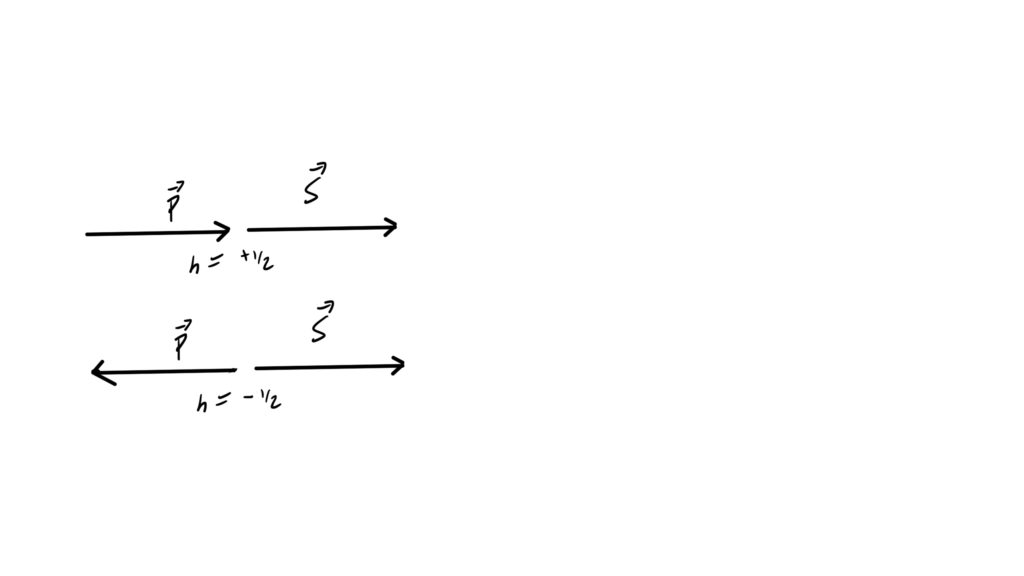## PHY2403H Quantum Field Theory. Lecture 20: Dirac Lagrangian, spinor solutions to the KG equation, Dirac matrices, plane wave solution, helicity. Taught by Prof. Erich Poppitz

[Here is another PDF only post, containing my notes for Lecture 20 of the UofT QFT I (quantum field theory) course.]

In this lecture Professor Poppitz derived a rest frame solution of the Dirac equation, then demonstrated that the generalization to non-zero momentum satisfied the equation. We also saw that Dirac spinor solutions of the Dirac equation are KG equation solutions, and touched on the relation of some solutions to the helicity operator.This post doesn’t have a web version, since my latex -> wordpress-mathjax script doesn’t have support for the theorem/lemma environments that I used for Monday’s notes, and I don’t have time to implement that right now.

## Reflection using Pauli matrices.

In class yesterday (lecture 19, notes not yet posted) we used $$\Bsigma^\T = -\sigma_2 \Bsigma \sigma_2$$, which implicitly shows that $$(\Bsigma \cdot \Bx)^\T$$ is a reflection about the y-axis.
This form of reflection will be familiar to a student of geometric algebra (see  — a great book, one copy of which is in the physics library). I can’t recall any mention of the geometrical reflection identity from when I took QM. It’s a fun exercise to demonstrate the reflection identity when constrained to the Pauli matrix notation.

## Theorem: Reflection about a normal.

Given a unit vector $$\ncap \in \mathbb{R}^3$$ and a vector $$\Bx \in \mathbb{R}^3$$ the reflection of $$\Bx$$ about a plane with normal $$\ncap$$ can be represented in Pauli notation as
\begin{equation*}
-\Bsigma \cdot \ncap \Bsigma \cdot \Bx \Bsigma \cdot \ncap.
\end{equation*}

To prove this, first note that in standard vector notation, we can decompose a vector into its projective and rejective components
\begin{equation}\label{eqn:reflection:20}
\Bx = (\Bx \cdot \ncap) \ncap + \lr{ \Bx – (\Bx \cdot \ncap) \ncap }.
\end{equation}
A reflection about the plane normal to $$\ncap$$ just flips the component in the direction of $$\ncap$$, leaving the rest unchanged. That is
\begin{equation}\label{eqn:reflection:40}
-(\Bx \cdot \ncap) \ncap + \lr{ \Bx – (\Bx \cdot \ncap) \ncap }
=
\Bx – 2 (\Bx \cdot \ncap) \ncap.
\end{equation}
We may write this in $$\Bsigma$$ notation as
\begin{equation}\label{eqn:reflection:60}
\Bsigma \cdot \Bx – 2 \Bx \cdot \ncap \Bsigma \cdot \ncap.
\end{equation}
We also know that
\begin{equation}\label{eqn:reflection:80}
\begin{aligned}
\Bsigma \cdot \Ba \Bsigma \cdot \Bb &= a \cdot b + i \Bsigma \cdot (\Ba \cross \Bb) \\
\Bsigma \cdot \Bb \Bsigma \cdot \Ba &= a \cdot b – i \Bsigma \cdot (\Ba \cross \Bb),
\end{aligned}
\end{equation}
or
\begin{equation}\label{eqn:reflection:100}
a \cdot b = \inv{2} \symmetric{\Bsigma \cdot \Ba}{\Bsigma \cdot \Bb},
\end{equation}
where $$\symmetric{\Ba}{\Bb}$$ is the anticommutator of $$\Ba, \Bb$$.
Inserting \ref{eqn:reflection:100} into \ref{eqn:reflection:60} we find that the reflection is
\begin{equation}\label{eqn:reflection:120}
\begin{aligned}
\Bsigma \cdot \Bx –
\symmetric{\Bsigma \cdot \ncap}{\Bsigma \cdot \Bx}
\Bsigma \cdot \ncap
&=
\Bsigma \cdot \Bx –
{\Bsigma \cdot \ncap}{\Bsigma \cdot \Bx}
\Bsigma \cdot \ncap

{\Bsigma \cdot \Bx}{\Bsigma \cdot \ncap}
\Bsigma \cdot \ncap \\
&=
\Bsigma \cdot \Bx –
{\Bsigma \cdot \ncap}{\Bsigma \cdot \Bx}
\Bsigma \cdot \ncap

{\Bsigma \cdot \Bx} \\
&=

{\Bsigma \cdot \ncap}{\Bsigma \cdot \Bx}
\Bsigma \cdot \ncap,
\end{aligned}
\end{equation}
which completes the proof.

When we expand $$(\Bsigma \cdot \Bx)^\T$$ and find
\begin{equation}\label{eqn:reflection:n}
(\Bsigma \cdot \Bx)^\T
=
\sigma^1 x^1 – \sigma^2 x^2 + \sigma^3 x^3,
\end{equation}
it is clear that this coordinate expansion is a reflection about the y-axis. Knowing the reflection formula above provides a rationale for why we might want to write this in the compact form $$-\sigma^2 (\Bsigma \cdot \Bx) \sigma^2$$, which might not be obvious otherwise.

# References

 C. Doran and A.N. Lasenby. Geometric algebra for physicists. Cambridge University Press New York, Cambridge, UK, 1st edition, 2003.

## Applied vanity press

Amazon’s createspace turns out to be a very cost effective way to get a personal color copy of large pdf (>250 pages) to markup for review. The only hassle was having to use their app to create cover art (although that took less time than commuting downtown to one of the cheap copy shops near the university.)

As a side effect, after I edit it, I’d have something I could actually list for sale.  Worldwide, I’d guess at least three people would buy it, that is, if they weren’t happy with the pdf version already available.

## Geometric algebra notes collection split into two volumes

I’ve now split my (way too big) Exploring physics with Geometric Algebra into two volumes:

Each of these is now a much more manageable size, which should facilitate removing the redundancies in these notes, and making them more properly book like.

Also note I’ve also previously moved “Exploring Geometric Algebra” content related to:

• Lagrangian’s
• Hamiltonian’s
• Noether’s theorem

into my classical mechanics collection (449 pages).